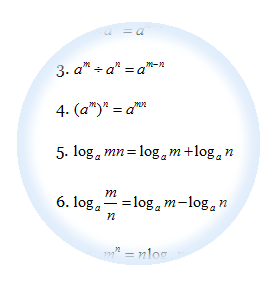# Formulae that will be given in SPM Add MathsThese are the formulae that will be given to help you to answer the SPM Additional Maths Questions. However, more important is that you know how to apply those formulaes to solve your questions. Buy some exercises from the bookstore and work on the questions everyday and you will master all those formulaes. Basically there are divided into 5 categories:

1. Algebra
2. Calculus
3. Statistics
4. Geometry
5. Trigonometry

``

This topic is closely related to the topic of quadratic equations. We will discuss further  on 4 subtopics below:

1. The Basic of quadratic functions
2. Determine max and min values of quadratic function
3. How to sketch the graph of quadratic functions
4. How to find the range of values of x in Quadratic inequalities

## 3 Basic Techniques in Solving Quadratic Equation Questions

In this chapter we will learn 3 most basic techniques on how to:

3. Determine the conditions for the type of roots.

# Functions

## 4  Basic Concepts You Need To Learn About Functions

Basically there are four concepts you need to master in this chapter:

1. Relations
2. Functions
3. Composite Functions
4. Inverse Functions